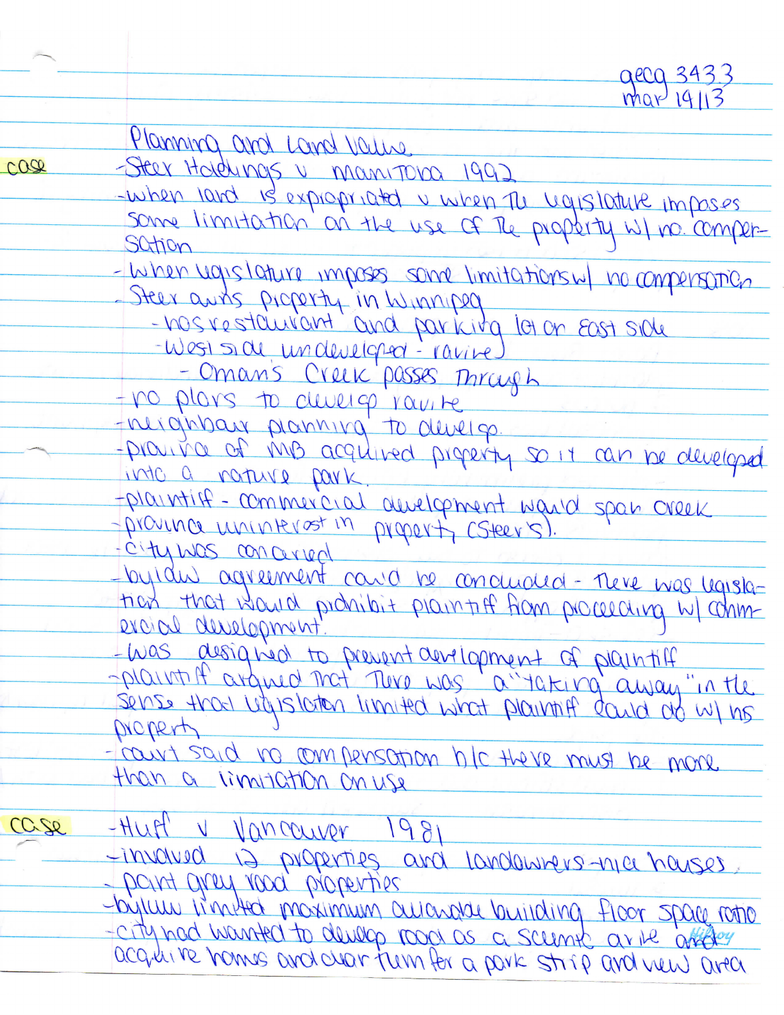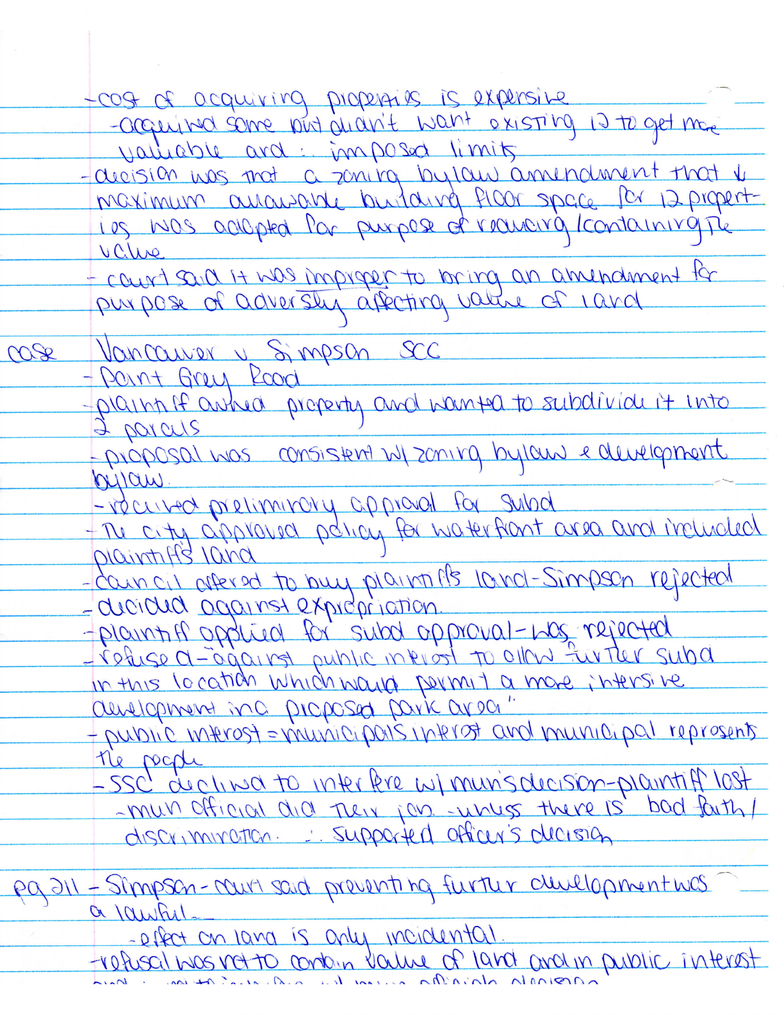Class Notes (1,100,000)
CA (630,000)
U of S (3,000)
PLAN (60)
Lecture

geog 343 march 14.pdf

Department
Planning
Course Code
PLAN 343
Professor
Maria Lynn Freeland

This preview shows page 1. to view the full 5 pages of the document.JI^D^t:&kX^^^^
.CtfY-
Q&^eL-aj^JuJXJ^
i
SlSd^O-tf*^
^^\aCV_J^JuJ
AO^OUspak
£v'?5^
vvy
n\C^ftt^_jCSfeLM-S-!
VM
2i!Mi^i_iX)s^L^
^jaiO^jm-^^^
lLOMk^4^i^^
Q-Jfc&g-
Jk^liXk^Ol_£U^
_^S^£LAiMMa<^
-
J
\
H^Ia
You're Reading a Preview

Unlock to view full version

Only page 1 are available for preview. Some parts have been intentionally blurred.^
£kkk0^^
J
|rcv\AC\V^VQ\k
__
_______
____
__
^
A\._
^^^-^^^i-.&^\t.
^^SSlA^^zS^^^lM^^SZ
is^ssLCLziy^j^^
^Uc^XA)c3u^i^^i-Ii^^
4^
SS&L-S^&ti^^
^
cyUv
\v\OAoyvA\cx\
LiX^iv^Mx^.
j£.A^XV^
_
1\
You're Reading a Preview

Unlock to view full version